# Test: Electrical Machines- 1

## 20 Questions MCQ Test Mock Test Series for SSC JE Electrical Engineering | Test: Electrical Machines- 1

Description
Attempt Test: Electrical Machines- 1 | 20 questions in 12 minutes | Mock test for SSC preparation | Free important questions MCQ to study Mock Test Series for SSC JE Electrical Engineering for SSC Exam | Download free PDF with solutions
QUESTION: 1

### In a DC machine, which of the following statement is true?

Solution:

The compensating windings consist of a series of coils embedded in slots in the pole faces. These coils are connected in series with the armature. The series-connected compensating windings produce a magnetic field, which varies directly with armature current. Because the compensating windings are wound to produce a field that opposes the magnetic field of the armature, they tend to cancel the cross magnetizing effect of the armature magnetic field.

Interpoles are similar to the main field poles and located on the yoke between the main field poles. They have windings in series with the armature winding. Interpoles have the function of reducing the armature reaction effect in the commutating zone. They eliminate the need to shift the brush assembly.

QUESTION: 2

### A dc shunt generator has full-load voltage regulation of 10% at rated speed of 1000 rpm. If it is now driven at 1250 rpm, then its voltage regulation at full load would

Solution: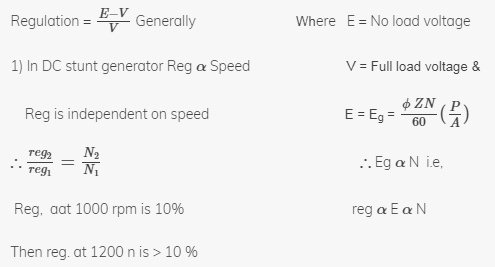QUESTION: 3

### The voltage applied to a transformer primary is increased by keeping v/f ratio constant, with this the core loss will

Solution:

As v/f ratio is constant.

If v increases, f also increases.

As frequency increases, core losses also increases.

We know that,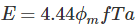As v and f both increases, flux remain constant and hence magnetizing current will remain constant.

QUESTION: 4

In a synchronous generator operating at zero power factor lagging. The effect of armature reaction is

Solution:

At lagging zero electrical power factor, the armature current lags by 90° to induced emf in the armature. As the emf induced in the armature coil due to main field flux thus the emf leads the main field flux by 90°.

Armature flux and field flux act directly opposite to each other. Hence armature reaction of the alternator at lagging zero power factor is a purely demagnetising type. That means, armature flux directly weakens main field flux.

QUESTION: 5

Skewing of the rotor in a three-phase squirrel-cage induction motor reduces

Solution:

If the rotor slots are skewed then the effect of slot harmonics (parasitic torques) can be eliminated.

Since the skewed rotor slot is not parallel to start slot in an induction motor, there is more leakage reactance. As a result, induction motor has lower starting and maximum torques.

With skewed rotor bars, the revolving flux, in effect, encounters an air gap of uniform reluctance and this results in a uniform torque and quieter operation.

QUESTION: 6

A series motor drawing an armature current of Ia is operating under saturated magnetic conditions. The torque developed in the motor is proportional to

Solution:

Torque developed in a series motor is,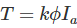As motor is working under magnetic saturated conditions, the flux is constant. Hence torque developed is directly proportional to armature current.

QUESTION: 7

Iron losses of transformer are measured by

Solution:

Iron losses of transformer are measured by conducting open circuit test. During open circuit test transformer works at very low power factor.

The low power factor wattmeter is the instrument that measures lower values of power factor accurately.

Hence low pf wattmeter is used to measure iron losses of transformer.

QUESTION: 8

In lap winding, the number of brushes is always

Solution:

In lap winding, the number of parallel paths = number of brushes = number of poles

In wave winding, the number of parallel paths = number of brushes = 2

QUESTION: 9

A transformer having 1200 primary turns is connected to a 240 V a.c. supply for a secondary voltage of 360 V, the number of turns on the secondary should be

Solution:

Given that, primary turns (N1) = 1200

Primary voltage (V1) = 240 V

Secondary voltage (V2) = 360 V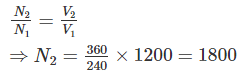QUESTION: 10

The maximum efficiency of a 100 kVA transformer having iron loss of 900 W and full load Cu loss of 1600 W occurs at________kVA.

Solution:

The maximum efficiency of a transformer occurs at

kVA at maximum efficiency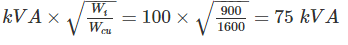QUESTION: 11

Torques developed by a split phase motor is proportional to

Solution:

A split-phase motor has no capacitance in the auxiliary circuit. A phase shift with respect to the main current is achieved by using narrow conductors to achieve a high resistance to reactance ratio.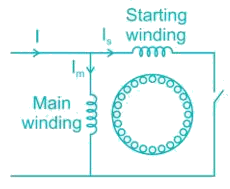Increasing the resistance means that the auxiliary winding can only be used during starting, otherwise, it would overheat.

A split-phase motor has significantly lower torque at starting than any of the capacitor motors due to the reduced phase angle between main and starting winding currents.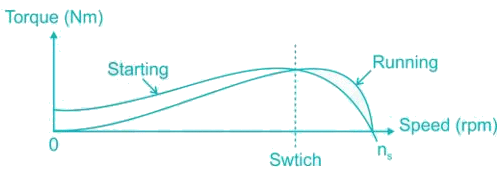Torques developed by a split phase motor is proportional to sine of angle between Im and Is

QUESTION: 12

A fluctuating voltage supply is detrimental to a refrigerator motor, but not a ceiling fan motor, although both are single-phase induction motors because, the refrigerator motor

Solution:

A fluctuating voltage supply is detrimental to a refrigerator motor, but not a ceiling fan motor because the refrigerator motor is enclosed in a sealed unit while the fan motor is open to the environment.

QUESTION: 13

Consider the following steps

1) Reversing connections to the terminals of the capacitor

2) Changing the position of the capacitor from auxiliary winding circuit to main winding circuit

3) Reversing supply connection to the main winding

4) Reversing supply connection to the auxiliary circuit

While installing a new ceiling fan, if the fan motor is found to be rotating in the wrong directions then the direction of rotation of fan motor can be corrected by

Solution:

The direction of rotation of fan motor can be corrected by
2) Changing the position of the capacitor from auxiliary winding circuit to main winding circuit.

3) Reversing supply connection to the main winding.
4) Reversing supply connection to the auxiliary circuit

QUESTION: 14

For a given output and speed, a universal motor as compared to 220 V, 50 Hz supply will require

Solution:

For a given output and speed, a universal motor as compared to 220 V, 50 Hz supply will require less voltage at low frequency.

QUESTION: 15

For domestic sewing machine, the size of motor required is

Solution:

Universal motors are used mostly in household sewing machines. The size of the motor is around 100 to 150 watts.

QUESTION: 16

In an induction motor, if the rotor resistance is equal to standstill reactance then the maximum torque is

Solution:

At maximum torque, rotor resistance is equal to slip times of reactance.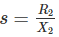At starting condition (s = 1), rotor resistance is equal to standstill reactance.

QUESTION: 17

The machine which is designed to run without an active load but with its excitation to produce reactive power is called

Solution:

Synchronous compensator is a DC-excited synchronous motor, whose shaft is not connected to anything but spin freely. Its purpose is not to convert electric power to mechanical power or vice versa, but to adjust conditions on the electric power transmission grid. Its field is controlled by a voltage regulator to either generate or absorb reactive power as needed to adjust the grid's voltage or to improve power factor.

QUESTION: 18

Reluctance torque in rotating machines is present when_______

Solution:

Metals like Iron provide a low reluctance path and the flux lines like to pass through the least reluctance path. The least reluctance path is along the pole axis where the air gap is minimum. So the flux lines will exert force on the rotor to align with the flux lines. This torque is created additional to the torque produced by the locking of excited poles with synchronously rotating magnetic field. This effect is absent in cylindrical rotor machine as the air gap is uniform.

QUESTION: 19

The squirrel cage winding of a single phase motor is placed in the

Solution:

Explanation : A synchronous motor may have a squirrel-cage winding embedded in its rotor, used to increase the motor starting torque and so decrease the time to accelerate to synchronous speed. The squirrel cage winding of a synchronous machine will generally be smaller than for an induction machine of similar rating. When the rotor is turning at the same speed as the stator's revolving magnetic field, no current is induced into the squirrel-cage windings and the windings will have no further effect on the operation of the synchronous motor at steady-state.

QUESTION: 20

Alternators are classified into

Solution:

Alternators are classified into salient pole and non-salient pole machines.

In salient pole type of rotor consist of large number of projected poles (salient poles) mounted on a magnetic wheel. Typically number of salient poles are 4 to 60. Salient pole rotors have large diameter and shorter axial length. Flux distribution is relatively poor than non-salient pole rotor, hence the generated emf waveform is not as good as cylindrical rotor.

Non-salient pole rotors are cylindrical in shape having parallel slots on it to place rotor windings. Number of poles is usually 2 or 4. They are smaller in diameter but having longer axial length. Flux distribution is sinusoidal and hence gives better emf waveform.Use Code STAYHOME200 and get INR 200 additional OFF Use Coupon Code